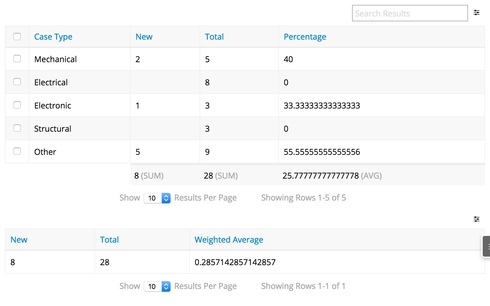# Displaying results from different model conditions in the same table.

Hi, all. I'm hoping you can help. I'm just starting to try to solve this problem and am researching the best approach. I'm building a table that I'm hoping to use to aggregate the results of different model conditions and so far what I'm coming up with seems needlessly complex.

What I'd like to display is:
`Requests  ||  New  ||  Total  || %  Service 1 ||    1  ||     10  || 10.0%  Service 2 ||    4  ||     16  || 25.0%  TOTAL     ||    5  ||     26  || 19.2%  `
﻿I built an aggregate model to show all requests that were received in the given time period and another to display all of them that were in New status. I don't know how to get them in the same row or add the percentage UI-Field formula so that it will perform the calculations on each row rather than overall.

• Hi Shane, if you are just looking to display the results, you may be able to use a template component, or template fields in a table. Global Merge Syntax will let you pull data from other models and fields and display it anywhere you want.

If your use case is more involved and requires that your aggregations are displayed in context of other objects, you may find this recent post involving UI-Only formula fields and the MODEL_LOOKUP function helpful as you look for a solution that works for you.
• Thanks for the heads up, Mark. I'll take a look at these references.
•The following is the XML to create the beautiful table that is shown above. Your data may vary based on your own org, but nothing on this page is custom.

This takes Groupings, Aggregate Models, Formula Fields, Models arranged in the right manner, PEMDAS, and patience to accomplish.

Both tables you’re looking at combine two models by using the MODEL_LOOKUP that Mark is referring too. They are robust and take some time to work through to understand.

Unfortunately, at this time, we are not able to call upon column summaries declaratively, nor are we able to manipulate them to make a weighted average like your post has indicated. Hopefully, we’ll be able to do this in the future. If it is something that interests you, write it up in the community as an idea and vote “me too” if it is posted below.

If you have any questions on how this was built, please ask.
• <skuidpage unsavedchangeswarning="yes" personalizationmode="server" showsidebar="false" useviewportmeta="true" showheader="false">
<models>
<model id="CaseAggregate" limit="" query="true" createrowifnonefound="false" datasource="salesforce" type="aggregate" sobject="Case" doclone="">
<fields>
<field id="Id" name="countId3000" function="COUNT"/>
<field id="Bringing_in_New_Status_From_CaseAggregate1" uionly="true" displaytype="FORMULA" ogdisplaytype="TEXT" readonly="true" returntype="DOUBLE" precision="9" scale="0">
<formula>MODEL_LOOKUP("CaseAggregate1","countId","type",{{type}})</formula>
</field>
<field id="Percentage" uionly="true" readonly="true" displaytype="FORMULA" ogdisplaytype="TEXT" returntype="TEXT">
<formula>{{Bringing_in_New_Status_From_CaseAggregate1}}/{{countId3000}}*100</formula>
</field>
</fields>
<conditions/>
<actions/>
<groupby method="simple">
<field id="Type" name="type"/>
</groupby>
</model>
<model id="CaseAggregate1" limit="" query="true" createrowifnonefound="false" datasource="salesforce" type="aggregate" sobject="Case" doclone="">
<fields>
<field id="Id" name="countId" function="COUNT"/>
<field id="Bringing_in_CaseAggregate" uionly="true" displaytype="FORMULA" ogdisplaytype="TEXT" readonly="true" returntype="DOUBLE" precision="9" scale="0">
<formula>MODEL_LOOKUP("CaseAggregate","countId3000","type",{{type}})</formula>
</field>
</fields>
<conditions>
<condition type="fieldvalue" value="New" enclosevalueinquotes="true" field="Status"/>
</conditions>
<actions/>
<groupby method="simple">
<field id="Type" name="type"/>
</groupby>
</model>
<model id="Summation_of_New_Status" limit="" query="true" createrowifnonefound="false" datasource="salesforce" type="aggregate" sobject="Case" doclone="">
<fields>
<field id="Status" name="countStatus_here" function="COUNT"/>
</fields>
<conditions>
<condition type="fieldvalue" value="New" enclosevalueinquotes="true" field="Status"/>
</conditions>
<actions/>
<groupby method="simple"/>
</model>
<model id="WeightedAverageModel" limit="" query="true" createrowifnonefound="false" datasource="salesforce" type="aggregate" sobject="Case">
<fields>
<field id="Status" name="countStatus" function="COUNT"/>
<field id="NewStatusCount" uionly="true" readonly="true" displaytype="FORMULA" ogdisplaytype="TEXT" returntype="TEXT" precision="9" scale="0">
<formula>{{\$Model.Summation_of_New_Status.data.0.countStatus_here}}</formula>
</field>
<field id="Weighted_Average_Final" uionly="true" readonly="true" displaytype="FORMULA" ogdisplaytype="TEXT" returntype="TEXT">
<formula>{{NewStatusCount}}/{{countStatus}}</formula>
</field>
</fields>
<conditions/>
<actions/>
<groupby method="simple"/>
</model>
</models>
<components>
<skootable showconditions="true" showsavecancel="false" showerrorsinline="true" searchmethod="server" searchbox="true" showexportbuttons="false" pagesize="10" createrecords="false" model="CaseAggregate" buttonposition="" mode="readonly" allowcolumnreordering="true" uniqueid="sk-2KjbZV-145">
<fields>
<field id="Type" name="type" hideable="true" uniqueid="fi-2KkdBe-196" valuehalign="" type=""/>
<field id="Bringing_in_New_Status_From_CaseAggregate1" name="Bringing_in_New_Status_From_CaseAggregate1" hideable="true" uniqueid="fi-2Lrt3b-6635" decimalplaces="" valuehalign="" type="">
<label>New</label>
<summaries>
<summary>sum</summary>
</summaries>
</field>
<field id="Id" name="countId3000" hideable="true" uniqueid="fi-2Kq-Z9-704" valuehalign="" type="">
<summaries>
<summary>sum</summary>
</summaries>
<label>Total</label>
</field>
<field id="Percentage" hideable="true" uniqueid="fi-2Kt5hH-1276" valuehalign="" type="">
<label>Percentage</label>
<summaries>
<summary>avg</summary>
</summaries>
</field>
</fields>
<rowactions/>
<massactions usefirstitemasdefault="true"/>
<views>
<view type="standard"/>
</views>
<renderconditions logictype="and"/>
<searchfields/>
</skootable>
<skootable showconditions="true" showsavecancel="false" showerrorsinline="true" searchmethod="server" searchbox="true" showexportbuttons="false" pagesize="10" createrecords="false" model="WeightedAverageModel" buttonposition="" mode="readonly" allowcolumnreordering="true" uniqueid="sk-2LSeTF-1827">
<fields>
<field id="NewStatusCount" name="NewStatusCount" hideable="true" uniqueid="fi-2LlJwN-5247" valuehalign="" type="">
<label>New</label>
</field>
<field id="Status" name="countStatus" hideable="true" uniqueid="fi-2Lle5o-5273" valuehalign="" type="">
<label>Total</label>
</field>
<field id="Weighted_Average_Final" hideable="true" uniqueid="fi-2LiUrV-4570" valuehalign="" type="">
<label>Weighted Average</label>
</field>
</fields>
<rowactions/>
<massactions usefirstitemasdefault="true"/>
<views>
<view type="standard"/>
</views>
</skootable>
</components>
<resources>
<labels/>
<javascript/>
<css/>
</resources>
<styles>
<styleitem type="background" bgtype="none"/>
</styles>
</skuidpage>